##### Calculus Essentials For Dummies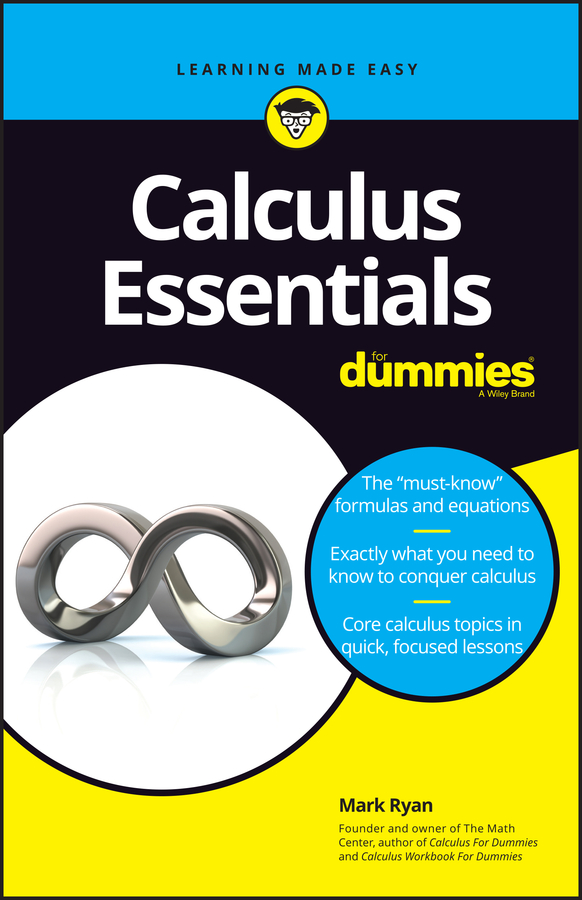In calculus, an infinite series is "simply" the adding up of all the terms in an infinite sequence. Despite the fact that you add up an infinite number of terms, some of these series total up to an ordinary finite number. Such series are said to converge. If a series doesn't converge, it's said to diverge. Whether a series converges or diverges is one of the first and most important things you will want to determine about the series.

Here's a look at various methods you can use to test the convergence or divergence of an infinite series.

• nth term test: If the nth term of a series doesn't converge to zero, the series diverges.

• Geometric series: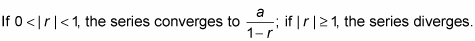• p-series: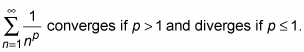• Ratio test: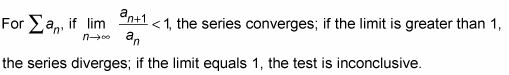• Root test: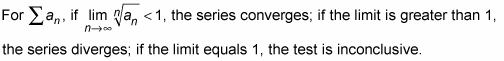• Direct comparison test: If the given series is smaller than your convergent benchmark series, then the given series converges as well; if the given series is larger than your divergent benchmark series, then the given series diverges as well.

• Integral comparison test: If the benchmark improper integral converges, so does the given series; ditto for divergence.

• Limit comparison test: For two series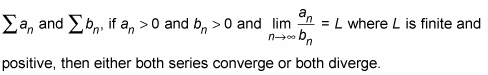• Alternating series test: An alternating series converges if

1. Its nth term converges to zero.

2. Each term is less than or equal to the preceding term (ignoring the negative signs).